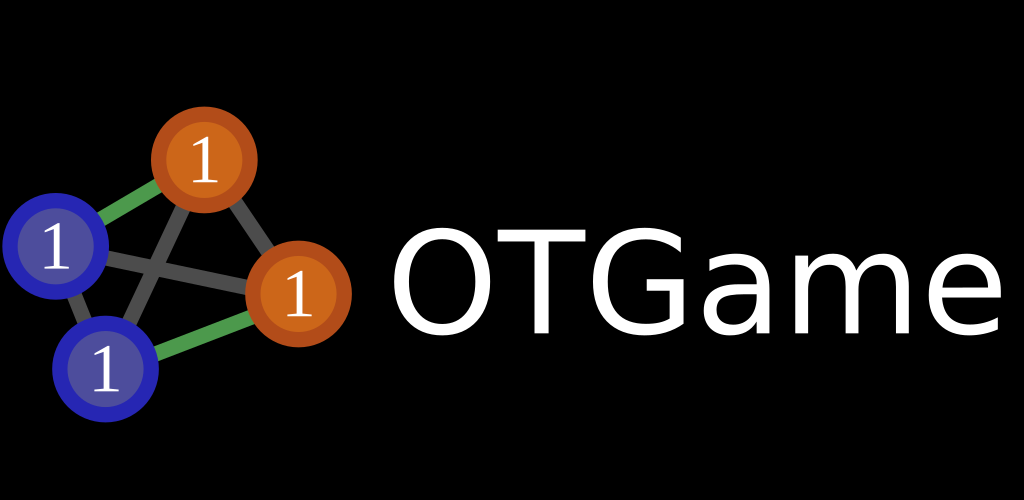# Rémi Flamary

Professional website

## Software

Here you can find a list of all the software and code that is available on my website. If you use it in your research, please cite the corresponding paper.

### POT: Python Optimal Transport libraryPOT is a Python library for Optimal Transport providing several numerical solvers for Optimal Transport and its extensions. I am the co-created of this library with Nicolas Courty. POT has been published in the JMLR Open Source Software track in 2021. In June 2023, POT was cited more than 500 times according to Google Scholar.

The toolbox is available on GitHub and we provide a documentation with several examples.

### OTGameOTGame is a game for Android that I developed where the objective is to solve discrete optimal transport problems visually with some help when finding a bad solution. It is provided with a video tutorial introducing the concept of OT and the gameplay. The game is available on the Google Play Store.

### Software and code from publications

I am a big fan of open science and reproducible research. I try to share the implementation of all my papers. You can find the code and data for most of my papers below and in the Publications page of the website.

• Time Series Alignment with Global Invariances [Code] [PDF]
• Template based Graph Neural Network with Optimal Transport Distances [Code] [PDF]
• Aligning individual brains with Fused Unbalanced Gromov-Wasserstein [Code] [PDF]
• Learning to Predict Graphs with Fused Gromov-Wasserstein Barycenters [Code] [PDF]
• Multi-source Domain Adaptation via Weighted Joint Distributions Optimal Transport [Code] [PDF]
• Sliding window strategy for convolutional spike sorting with Lasso: Algorithm, theoretical guarantees and complexity [Code] [PDF]
• Feature selection for kernel methods in systems biology [Code] [PDF]
• Convergent Working Set Algorithm for Lasso with Non-Convex Sparse Regularizers [Code] [PDF]
• Semi-relaxed Gromov Wasserstein divergence with applications on graphs [Code] [PDF]
• Unbalanced Optimal Transport through Non-negative Penalized Linear Regression [Code] [PDF]
• Optimal Transport for Conditional Domain Matching and Label Shift [Code] [PDF]
• Online Graph Dictionary Learning [Code] [PDF]
• Unbalanced minibatch Optimal Transport; applications to Domain Adaptation [Code] [PDF]
• POT: Python Optimal Transport [Code] [PDF]
• Generating natural adversarial Remote Sensing Images [Code] [PDF]
• Wasserstein Adversarial Regularization for learning with label noise [Code] [PDF]
• CO-Optimal Transport [Code] [PDF]
• Fused Gromov-Wasserstein Distance for Structured Objects [Code] [PDF]
• Sliced Gromov-Wasserstein [Code] [PDF]
• Influence of surface roughness on diffraction in the externally occulted Lyot solar coronagraph [Code] [PDF]
• Optimal Transport for Multi-source Domain Adaptation under Target Shift [Code] [PDF]
• DeepJDOT: Deep Joint distribution optimal transport for unsupervised domain adaptation [Code] [PDF]
• Large-Scale Optimal Transport and Mapping Estimation [Code] [PDF]
• Learning Wasserstein Embeddings [Code] [PDF]
• Wasserstein Discriminant Analysis [Code] [PDF]
• Joint Distribution Optimal Transportation for Domain Adaptation [Code] [PDF]
• Astronomical image reconstruction with convolutional neural networks [Code] [PDF]
• Performance of hybrid externally occulted Lyot solar coronagraph, Application to ASPIICS [Code] [PDF]
• Optimal spectral transportation with application to music transcription [Code] [PDF]
• Mapping estimation for discrete optimal transport [Code] [PDF]
• Optimal transport for domain adaptation [Code] [PDF]
• Doubly partial-diffusion LMS over adaptive networks [Code] [PDF]
• Supervised planetary unmixing with optimal transport [Code] [PDF]
• Introduction to optimization with applications in astronomy and astrophysics [Code] [PDF]
• Apprentissage statistique pour les BCI [Code] [PDF]
• Statistical learning for BCIs [Code] [PDF]
• Non-convex regularization in remote sensing [Code] [PDF]
• DC Proximal Newton for Non-Convex Optimization Problems [Code] [PDF]
• Multiclass feature learning for hyperspectral image classification: sparse and hierarchical solutions [Code] [PDF]
• A group-lasso active set strategy for multiclass hyperspectral image classification [Code] [PDF]
• Domain adaptation with regularized optimal transport [Code] [PDF]
• Mixed-Norm Regularization for Brain Decoding [Code] [PDF]
• Kernel-Based Learning From Both Qualitative and Quantitative Labels: Application to Prostate Cancer Diagnosis Based on Multiparametric MR Imaging [Code] [PDF]
• Automatic Feature Learning for Spatio-Spectral Image Classification With Sparse SVM [Code] [PDF]
• Nonconvex Regularizations for Feature Selection in Ranking With Sparse SVM [Code] [PDF]
• Learning with infinitely many features [Code] [PDF]
• Large Margin Filtering [Code] [PDF]
• Handling learning samples uncertainties in SVM : application to MRI-based prostate cancer Computer-Aided Diagnosis [Code] [PDF]
• lp-lq penalty for sparse linear and sparse multiple kernel multi-task learning [Code] [PDF]
• Handling uncertainties in SVM classification [Code] [PDF]
• Large margin filtering for signal sequence labeling [Code] [PDF]
• Filtrage vaste marge pour l'étiquetage séquentiel de signaux [Code] [PDF]
• Learning spatial filters for multispectral image segmentation [Code] [PDF]
• Selection de variables pour l'apprentissage simultanée de tâches [Code] [PDF]

### Older research related toolboxes and links

• POT, Python Optimal Transport library (Python).
• DWE, Deep Wasserstein Embeddings (Python).
• JDOT, Joint distribution optimal transportation for domain adaptation (Python).
• AstroImageReconsCNN, Astronomical Image Reconstruction with Convolutional Neural Networks (Python).
• OST, Optimal Spectral Transportation, musical annotation demo (Python).
• Opt. Non-convex, Optimization with non-convex regularization (Matlab/octave).
• OptTransp, Optimal transport toolbox with class regularization (Python).
• FL-RS-SVM, Feature learning for remote sensing (Matlab).
• IFL-SVM, Learning with an infinite number of features (Matlab).
• RankSVM-NC, RankSVM solver with non-convex regularization (Matlab).
• G-SVM, Linear SVM with general regularization (Matlab).
• FilterSVM, Learning large margin convolutional filters (Matlab).
• SparseMTL Sparse kernel multitask learning (Matlab).
• USVM Probabilistic SVM learning with uncertain labels (Matlab).
• Monqpcpp Quadratic Programming problem solver (C++).

### General purpose sofware

• SimpleSVN scripts GUI scripts to use subversion (tortoise like).
• Webgen.py Python implementation of the webgen website generator.